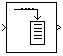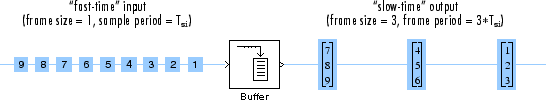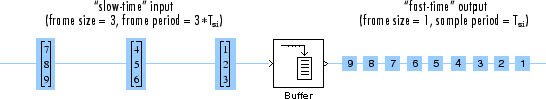# Buffer

Buffer input sequence to smaller or larger frame size

• Library:
• DSP System Toolbox / Signal Management / Buffers

•## Description

The Buffer block always performs frame-based processing. The block redistributes the data in each column of the input to produce an output with a different frame size. Buffering a signal to a larger frame size yields an output with a slower frame rate than the input. For example, consider this illustration for a scalar input.Buffering a signal to a smaller frame size yields an output with a faster frame rate than the input. For example, consider this illustration of a scalar output.The block coordinates the output frame size and frame rate of nonoverlapping buffers such that the sample period of the signal is the same at both the input and output: Tso = Tsi.

This block supports triggered subsystems when the block input and output rates are the same.

## Ports

### Input

expand all

To buffer single-channel signals, input a scalar or column vector. To buffer multichannel signals, input a row vector or matrix. To buffer single-channel signals, input a scalar or column vector of the size Mi-by-1, where Mi is the input frame size.

Data Types: `single` | `double` | `int8` | `int16` | `int32` | `uint8` | `uint16` | `uint32` | `Boolean` | `fixed point`
Complex Number Support: Yes

### Output

expand all

The buffered input signal returned as a vector or a matrix.

When the input is a single-channel signal:

• 1-by-1 (scalar) — Output is an Mo-by-1 vector.

• Mi-by-1 (column vector) — Output is an Mo-by-1 vector.

where

• Mi — Input frame size

• Mo — Value of the Output buffer size parameter

The input frame period is Mi · Tsi, where Tsi is the input sample period. The output frame period is $\left({M}_{o}-L\right){T}_{si}$, where L is the value of the Buffer overlap parameter and Tsi is the input sample period. When you set the Buffer overlap parameter to Mo – 1, the output frame period equals the input sample period.

When the input is a multichannel signal:

• 1-by-N — Output is an Mo-by-N matrix.

• Mi-by-N — Output is an Mo-by-N matrix.

where

• N — Number of channels in the signal

• Mi — Input frame period

• Mo is the value of the Output buffer size parameter and can be greater or less than the input frame size, Mi. The block buffers each of the N input channels independently.

The input frame period is Mi · Tsi. The output frame period is $\left({M}_{o}-L\right){T}_{si}$, which equals the sequence sample period when the Buffer overlap is ${M}_{o}-1$. Thus, the output sample period Tso is related to the input sample period Tsi by

`${T}_{so}=\frac{\left({M}_{o}-L\right){T}_{si}}{{M}_{i}}$`

The output has the same data type and complexity as the input

Data Types: `single` | `double` | `int8` | `int16` | `int32` | `uint8` | `uint16` | `uint32` | `Boolean` | `fixed point`
Complex Number Support: Yes

## Parameters

expand all

Specify the number of consecutive samples, Mo, from each channel to buffer into the output frame.

Data Types: `double` | `int8` | `int16` | `int32` | `uint8` | `uint16` | `uint32`

Specify the number of samples, L, which specifies the amount of overlap or underlap in each successive output frame.

To overlap the data in the buffer, specify a value of L in the range $0\le L<{M}_{o}$, where Mo is the value of the Output buffer size parameter. The block takes L samples (rows) from the current output and repeats them in the next output. In cases of overlap, the block acquires ${M}_{o}-L$new input samples before propagating the buffered data to the output.

When $L<0$, you are buffering the signal with underlap. The block discards L input samples after the buffer fills and outputs the buffer with period $\left({M}_{o}-L\right){T}_{si}$, which is longer than in the zero-overlap case.

The output frame period is $\left({M}_{o}-L\right){T}_{si}$, which equals the input sequence sample period, Tsi, when the Buffer overlap is ${M}_{o}-1$.

Data Types: `double` | `int8` | `int16` | `int32` | `uint8` | `uint16` | `uint32`

Specify the value of the block's initial output, in cases of nonzero latency, as a scalar, vector, or matrix.

For all cases of single-tasking operation (Simulink Coder) other than those listed in Zero-Tasking Latency, the Buffer block's buffer is initialized to the value(s) specified by the Initial conditions parameter. The block reads from this buffer to generate the first D output samples, where

`$D=\left\{\begin{array}{cc}{M}_{o}+L& \left(L\ge 0\right)\\ {M}_{o}& \left(L<0\right)\end{array}$`

The dimensions of the Initial conditions parameter depend on the Buffer overlap, L, and whether the input is single-channel or multichannel:

• When $L\ne 0$, the Initial conditions parameter must be a scalar.

• When $L=0$, the Initial conditions parameter can be a scalar, or it can be a vector with either of these constraints:

• For single-channel inputs, the Initial conditions parameter can be a vector of length Mo if Mi is 1, or a vector of length Mi if Mo is 1.

• For multichannel inputs, the Initial conditions parameter can be a vector of length Mo * N if Mi is 1, or a vector of length Mi * N if Mo is 1.

For all multitasking operations (Simulink Coder), use the `rebuffer_delay` function to compute the exact delay in samples that the Buffer block introduces for a given combination of buffer size and buffer overlap.

For general buffering between arbitrary frame sizes, the Initial conditions parameter must be a scalar, which is then repeated across all elements of the initial output(s). However, in the special case where the input is a 1-by-N row vector, and the output of the block is an Mo-by-N matrix, Initial conditions can be:

• An Mo-by-N matrix

• A length-Mo vector to be repeated across all columns of the initial output(s)

• A scalar to be repeated across all elements of the initial output(s)

In the special case where the output is a 1-by-N row vector, which is the result of unbuffering an Mi-by-N matrix, the Initial conditions can be:

• A vector containing Mi samples to output sequentially for each channel during the first Mi sample times

• A scalar to be repeated across all elements of the initial output(s)

For more information on latency and the Simulink® tasking modes, see Excess Algorithmic Delay (Tasking Latency) and Time-Based Scheduling and Code Generation (Simulink Coder).

Data Types: `single` | `double` | `int8` | `int16` | `int32` | `uint8` | `uint16` | `uint32` | `Boolean` | `fixed point`
Complex Number Support: Yes

## Block Characteristics

 Data Types `Boolean` | `double` | `fixed point` | `integer` | `single` Direct Feedthrough `no` Multidimensional Signals `no` Variable-Size Signals `no` Zero-Crossing Detection `no`

expand all

## Version History

Introduced before R2006a